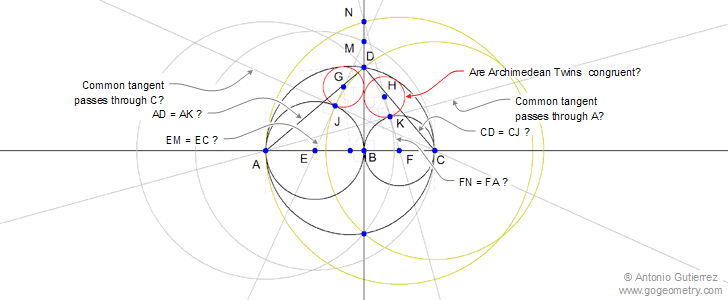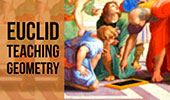# Geometry Expressions Symbolic System Constructions - Recent AdditionsI have used Geometry Expressions to visualize the Archimedean Twins above and check out a variety of conjectures. Geometry Expressions is the world's first Interactive Symbolic Geometry System. This means: Geometric figures can be defined by either Symbolic Constraints or numeric locations.

You can download Geometry Expressions 2 Free Trial. It is a fully featured 30 day evaluation copy of the software. All constraints, constructions and measurements are available for you to use.Geometry Expressions Help. Interactive Mind Map.

Sangaku Geometry Theorem: Cyclic Quadrilateral. Inradii, Rectangle.

Apollonius' Problem for Three Circles Illustration with animation and sound.

Seven Circles Theorem.
Step by Step illustration of this beautiful theorem.

Proposed Problem 57: Angle bisector, circles Cyclic Quadrilateral.

Proposed Problem 56: Angle bisector, circles Parallel Lines.

Proposed Problem 55: Angle bisector, circles Cyclic Quadrilateral.

Proposed Problem 54: Angle bisector, circles Midpoint of arc.

Sangaku Proposed Problem 41. "Mickey Mouse."
Three circles, tangents, radii.

 Home| Go Geometry Education | Search | Geometry | Arbelos | Semicircle | Email | By Antonio Gutierrez